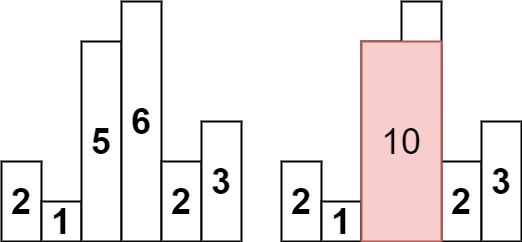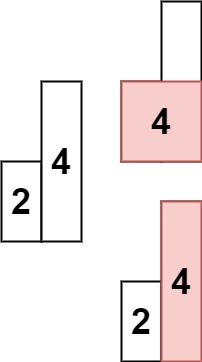# 84. Largest Rectangle in Histogram | LeetCode Solution

## 84. Largest Rectangle in Histogram | LeetCode Solution

Given an array of integers `heights` representing the histogram's bar height where the width of each bar is `1`, return the area of the largest rectangle in the histogram.

Example 1:```Input: heights = [2,1,5,6,2,3]
Output: 10
Explanation: The above is a histogram where width of each bar is 1.
The largest rectangle is shown in the red area, which has an area = 10 units.
```

Example 2:```Input: heights = [2,4]
Output: 4
```

Constraints:

• `1 <= heights.length <= 105`
• `0 <= heights[i] <= 104`

class Solution
{
public:
int largestRectangleArea(vector<int> &v)
{
stack<int> st;
int n = v.size();
int ans = 0;

for (int i = 0; i <= n; i++)
{
while (!st.empty() && (i == n || v[st.top()] >= v[i]))
{
int height = v[st.top()];
int width;
st.pop();
if (st.empty())
width = i;
else
width = i - st.top() - 1;
ans = max(ans, height * width);
}
st.push(i);
}
return ans;
}
};